# Introduction

## Purpose

This vignette shows you the main functionalities of the ‘ir’ package. This includes data import, functions for spectral preprocessing, and plotting.
This vignette does not explain the data structure of ir objects (the objects the package ir uses to store spectra) in detail and it does not describe general data manipulation functions (e.g. subsetting rows or columns, modifying variables) (for this, see vignette Introduction to the irclass). Moreover, this vignette does not explain the purpose of the spectral preprocessing functions.

## Structure

The vignette has three parts:

1. Data import and export
2. Plotting spectra
3. Spectral preprocessing

In part Data import and export, I will show how spetra can be imported from csv files and from Thermo Galactic’s spectral files (file extension .spc). I will also show how ir objects can be exported as csv files. To this end, I will use sample data which comes along with the ‘ir’ package. In part Plotting spectra, I will show how spectra can be plotted and how these plots can be modified.
In part Spectral preprocessing I will demonstrate the main preprocessing functions included in the ‘ir’ package and how these can be combined to execute complex preprocessing pipelines.

## Preparation

To follow this vignette, you have to install the ‘ir’ package as described in the Readme file and you have to load it:

library(ir)

# Data import and export

## Data import

To test importing spectra from files, I’ll use sample data which is contained in the ‘ir’ package (in folder inst/extdata). First, I’ll show how to import spectra from csv files and then how to import Thermo Galactic’s spectral files (file extension .spc).

### csv files

Spectra from csv files can be imported with ir_import_csv(). This function can import spectra from one or more csv files with the format shown here:

wavenumber GN.11.389 GN.11.400 GN.11.407 GN.11.411
4000 0.0003612 0.0001991 0.0001044 0.0001983
3999 0.0004313 0.0003787 0.0002027 0.0002307
3998 0.0005014 0.0005583 0.0003203 0.0002631
3997 0.0005712 0.0007378 0.0003938 0.0002954
3996 0.0006667 0.0009148 0.0004075 0.0003405
3995 0.0007045 0.0009870 0.0004077 0.0003683

This is a subset of the data we will import in a few moments. The first column must contain spectral channel values (“x axis values”, e.g. wavenumbers for mid infrared spectra), and each additional column represents the intensity values (“y axis values”, e.g. absorbances) of one spectrum. In the example above, there are four spectra in the csv file.

Then, you can simply pass the path to the file to ir_import_csv() and this will import the spectra:

d_csv <- ir_import_csv("../inst/extdata/klh_hodgkins_mir.csv", sample_id = "from_colnames")

The argument sample_id = "from_colnames" tells ir_import_csv() to extract names for the spectra from the column names of the csv file.

If you have additional metadata available, you can bind these to the ir object in a second step (note: here, I use functions from [dplyr] to reformat the metadata; you don’t need to understand the details of this data cleanup):

library(dplyr)
library(stringr)

as.is = TRUE) %>%
dplyr::rename(
sample_id = "Sample.Name",
sample_type = "Category",
comment = "Description",
holocellulose = "X..Cellulose...Hemicellulose..measured.",
klason_lignin = "X..Klason.lignin..measured."
) %>%
# make the sample_id values fir to those in d_csv\$sample_id to make combining easier
dplyr::mutate(
sample_id =
sample_id %>%
stringr::str_replace_all(pattern = "( |-)", replacement = "\\.")
)

d_csv <-
d_csv %>%
dplyr::full_join(d_csv_metadata, by = "sample_id")

Now, d_csv has addition columns with the added metadata.

### Thermo Galactic’s spc files

Spectra from spc files can be imported with ir_import_spc(). This function can import spectra from one or more spc files:

d_spc <- ir_import_spc("../inst/extdata/1.spc", log.txt = FALSE)

In this case, names for the spectra and other metadata are extracted from the spc file(s) and added to the ir object. You can inspect d_spc to see these additional variables.

## Data export

Data in ir objects can in principle be exported in many ways. Here, I show hot to export to a csv file with the same format as the sample data we imported in subsection csv files.

To this end, we first have to “flatten” the spectra column in ir_sample_data (using ir_flatten()) and export this as csv file using write.csv(). Second, to export the metadata, we have to drop the spectra from ir_sample_data (using ir_drop_spectra()), and then write the remaining data to a separate csv file using write.csv():

# export only the spectra
ir_sample_data %>%
ir_flatten() %>%
write.csv(tempfile("ir_sample_data_spectra", fileext = "csv"), row.names = FALSE)

ir_sample_data %>%
ir_drop_spectra() %>%
write.csv(tempfile("ir_sample_data_metadata", fileext = "csv"), row.names = FALSE)

# Plotting spectra

The ‘ir’ package provides a function to create simple plots of spectra out-of-the box:

plot(d_csv)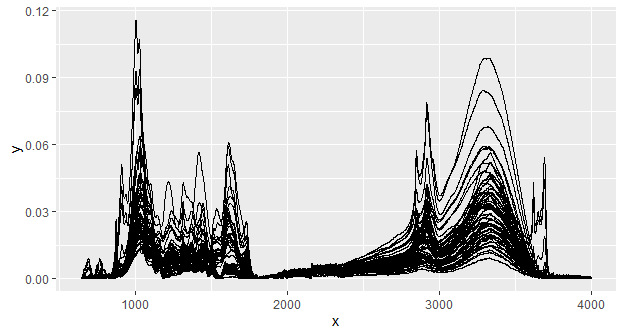This will plot the intensity values (“y axis values”, e.g. absorbances) of each spectrum versus the spectral channel values (“x axis values”, e.g. wavenumbers), connected by a line. All spectra in an ir object are plotted on top of each other.

ir relies on ggplot. This makes it possible to modify the plot with the functions from ggplot2. For example, we could color spectra according to the sample class:

library(ggplot2)

plot(d_csv) +
geom_path(aes(color = sample_type))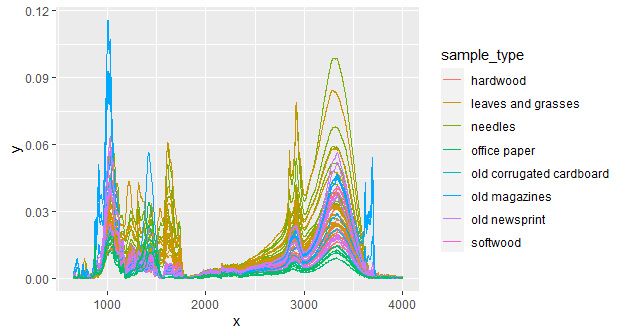An of course, we can change axis labels, layout, etc:

plot(d_csv) +
geom_path(aes(color = sample_type)) +
labs(x = expression("Wavenumber ["*cm^{-1}*"]"), y = "Absorbance") +
guides(color = guide_legend(title = "Sample type")) +
theme(legend.position = "bottom")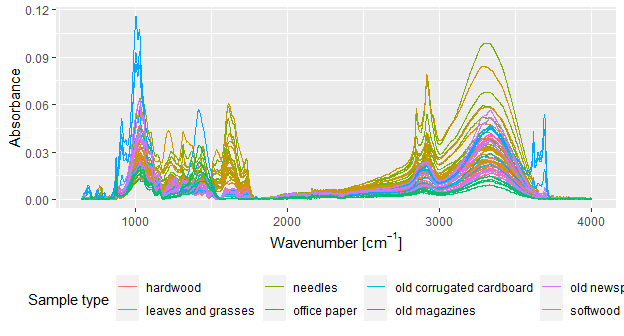# Spectral preprocessing

ir provides many functions for spectral preprocessing. Here, I’ll show how to use a subset of them. To make it easier to compare the effect, I’ll show here how the sample spectrum looks before any preprocessing:

plot(d_spc)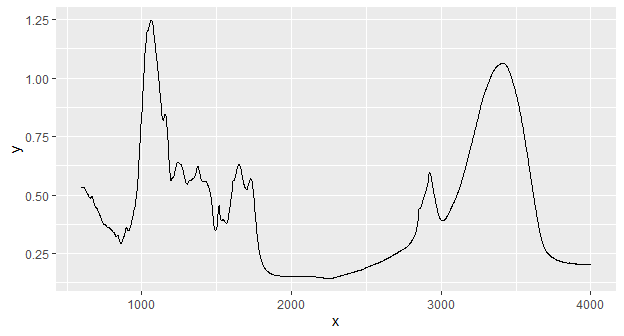## Baseline correction

Baseline correction with a rubberband algorithm (see the spc.rubberband function in the hyperspec package):

d_spc %>%
ir_bc(method = "rubberband") %>%
plot()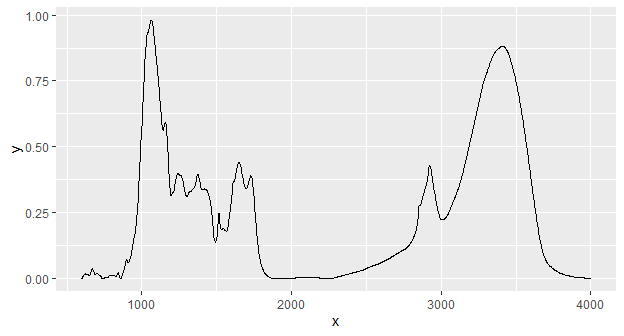## Normalization

Normalization of intensity values by dividing each intensity value by the sum of all intensity values (note the different scale of the y axis in comparison to the spectrum before preprocessing):

d_spc %>%
ir_normalize(method = "area") %>%
plot()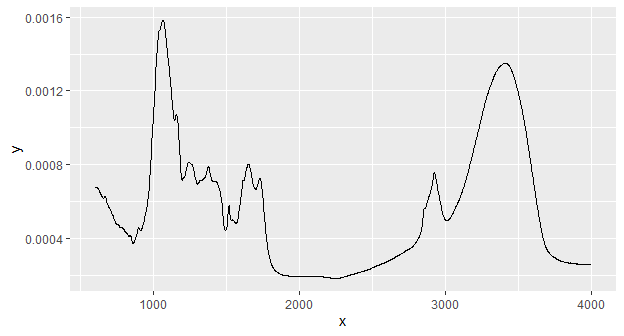Normalization of intensity values by dividing each intensity value by the the intensity value at a specific wavenumber (the horizontal and vertical lines highlight that the intensity at the selected wavenumber is 1 after normalization):

d_spc %>%
ir_normalize(method = 1090) %>%
plot() +
geom_hline(yintercept = 1, linetype = 2) +
geom_vline(xintercept = 1090, linetype = 2)
#> Warning: 1089.59485352039 selected instead of 1090.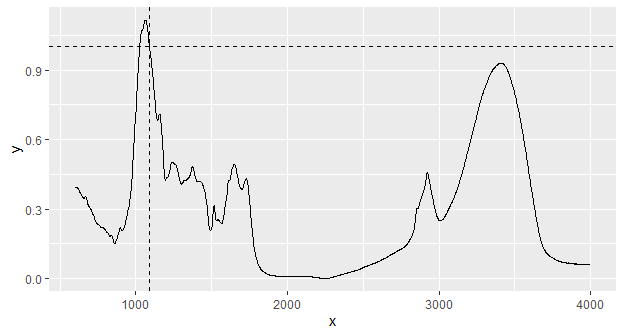The warning just says that the spectrum’s wavenumber values did not exactly match the desired value and therefore the nearest value available was selected. To disable this warning, you can interpolate the spectrum appropriately (see below, section Interpolating).

## Smoothing

Smoothing of spectra with the Savitzky-Golay algorithm (see the sgolayfilt() function from the signal package for details):

d_spc %>%
ir_smooth(method = "sg", p = 3, n = 91, m = 0) %>%
plot()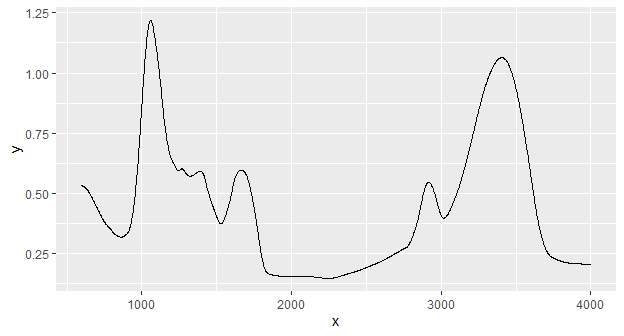## Derivative spectra

Savitzky-Golay smoothing can also be used to compute derivative spectra (here the first derivative is computed by setting the argument m to 1. See ?ir_smooth for more information):

d_spc %>%
ir_smooth(method = "sg", p = 3, n = 9, m = 1) %>%
plot()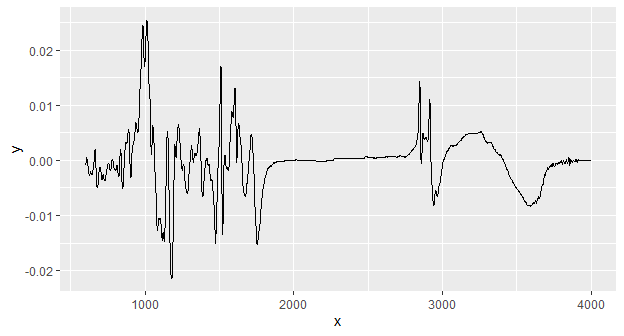## Clipping

Spectra can be clipped to desired ranges for spectral channels (“x axis values”, e.g. wavenumbers). Here, I clip the spectrum to the range [1000, 3000]:

d_spc %>%
ir_clip(range = data.frame(start = 1000, end = 3000)) %>%
plot()
#> Warning: 1000.88447606564 selected instead of 1000.
#> • 3000.7249417305 selected instead of 3000.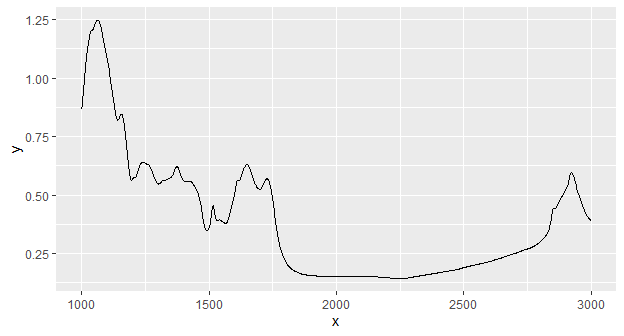## Interpolating

Spectral interpolation (interpolating intensity values for new wavenumber values) can be performed. Here, intensity values are interpolated for integer wavenumbers increasing by 1 (by setting dw = 1) within the range of the data:

d_spc %>%
ir_interpolate(dw = 1) %>%
plot()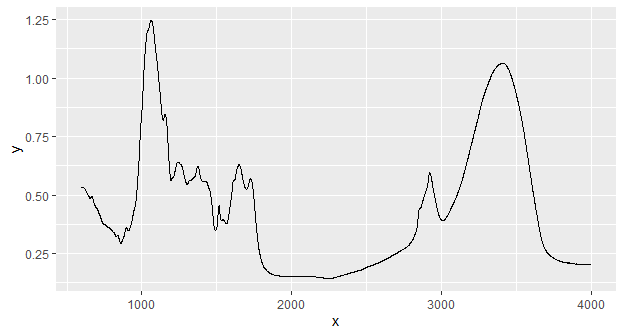This is not easy to see from the plot, but the warning shown above (section Normalization) during normalization now does not appear:

d_spc %>%
ir_interpolate(dw = 1) %>%
ir_normalize(method = 1090) %>%
plot() +
geom_hline(yintercept = 1, linetype = 2) +
geom_vline(xintercept = 1090, linetype = 2)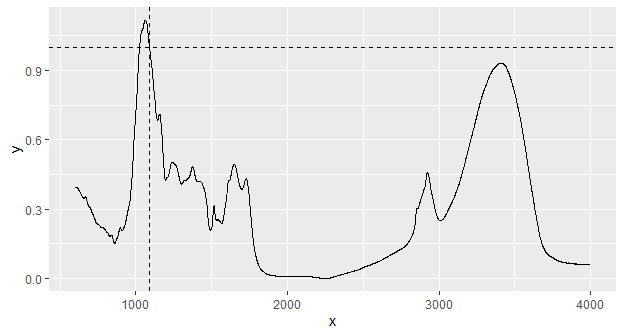## Interpolating regions

Sometimes, it is useful to replace parts of spectra by straight lines which connect the start and end points of a specified range. This can be done with ir_interpolate_region():

d_spc %>%
ir_interpolate_region(range = data.frame(start = 1000, end = 3000)) %>%
plot()
#> Warning: 1000.88447606564 selected instead of 1000.
#> • 3000.7249417305 selected instead of 3000.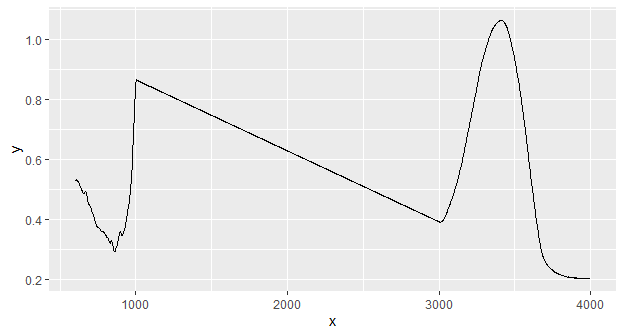## Binning

Spectral binning collects all intensity values in contiguous spectral ranges (“bins”) with specified widths and averages these:

d_spc %>%
ir_bin(width = 30) %>%
plot()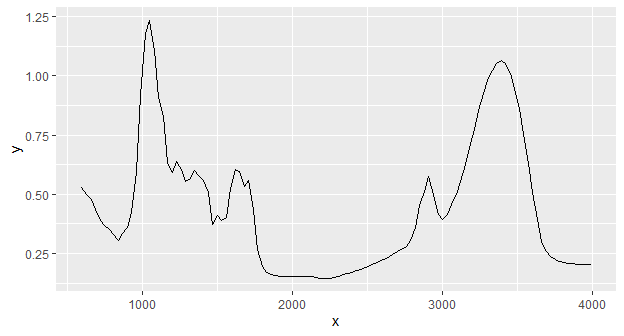## Building preprocessing pipelines

With ir, it is very easy to build complex reprocessing workflows by “piping” (using magrittr’s pipe (%>%) operator) together different preprocessing steps:

d_spc %>%
ir_interpolate(dw = 1) %>%
ir_clip(range = data.frame(start = 700, end = 3900)) %>%
ir_bc(method = "rubberband") %>%
ir_normalise(method = "area") %>%
ir_bin(width = 10) %>%
plot()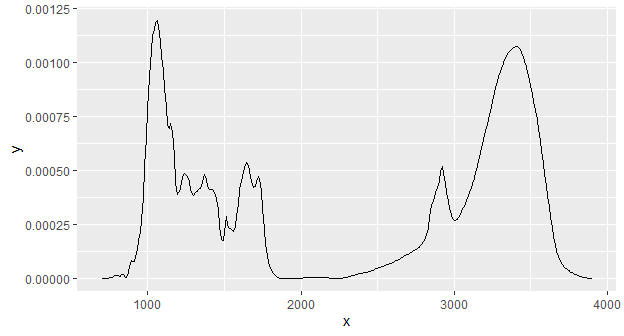Now, we have a baseline corrected spectrum, "area" normalized, clipped to [650, 3900], and binned to bin widths of 10 cm$$^{-1}$$.

# Further information

Many more functions and options to handle and process spectra are available in the ‘ir’ package. These are described in the documentation. In the documentation, you can also read more details about the functions and options presented here.
To learn more about the structure and general functions to handle ir objects, see the vignette Introduction to the irclass.

# Sources

The data contained in the csv file used in this vignette are derived from Hodgkins et al. (2018)

# Session info

#> R version 4.2.0 (2022-04-22 ucrt)
#> Platform: x86_64-w64-mingw32/x64 (64-bit)
#> Running under: Windows 10 x64 (build 22000)
#>
#> Matrix products: default
#>
#> locale:
#>  LC_COLLATE=C                    LC_CTYPE=German_Germany.utf8
#>  LC_MONETARY=German_Germany.utf8 LC_NUMERIC=C
#>  LC_TIME=German_Germany.utf8
#>
#> attached base packages:
#>  stats     graphics  grDevices utils     datasets  methods   base
#>
#> other attached packages:
#>  stringr_1.4.0    kableExtra_1.3.4 ggplot2_3.3.5    purrr_0.3.4
#>  dplyr_1.0.8      ir_0.2.1
#>
#> loaded via a namespace (and not attached):
#>   svglite_2.1.0       lattice_0.20-45     tidyr_1.2.0
#>   png_0.1-7           hyperSpec_0.100.0   digest_0.6.29
#>   utf8_1.2.2          R6_2.5.1            signal_0.7-7
#>  evaluate_0.15       httr_1.4.2          highr_0.9
#>  pillar_1.7.0        Rdpack_2.3          rlang_1.0.2
#>  lazyeval_0.2.2      rstudioapi_0.13     SparseM_1.81
#>  limSolve_1.5.6      jquerylib_0.1.4     rmarkdown_2.13
#>  labeling_0.4.2      webshot_0.5.3       munsell_0.5.0
#>  compiler_4.2.0      xfun_0.30           pkgconfig_2.0.3
#>  systemfonts_1.0.4   baseline_1.3-1      htmltools_0.5.2
#>  tidyselect_1.1.2    tibble_3.1.6        lpSolve_5.6.15
#>  sass_0.4.1